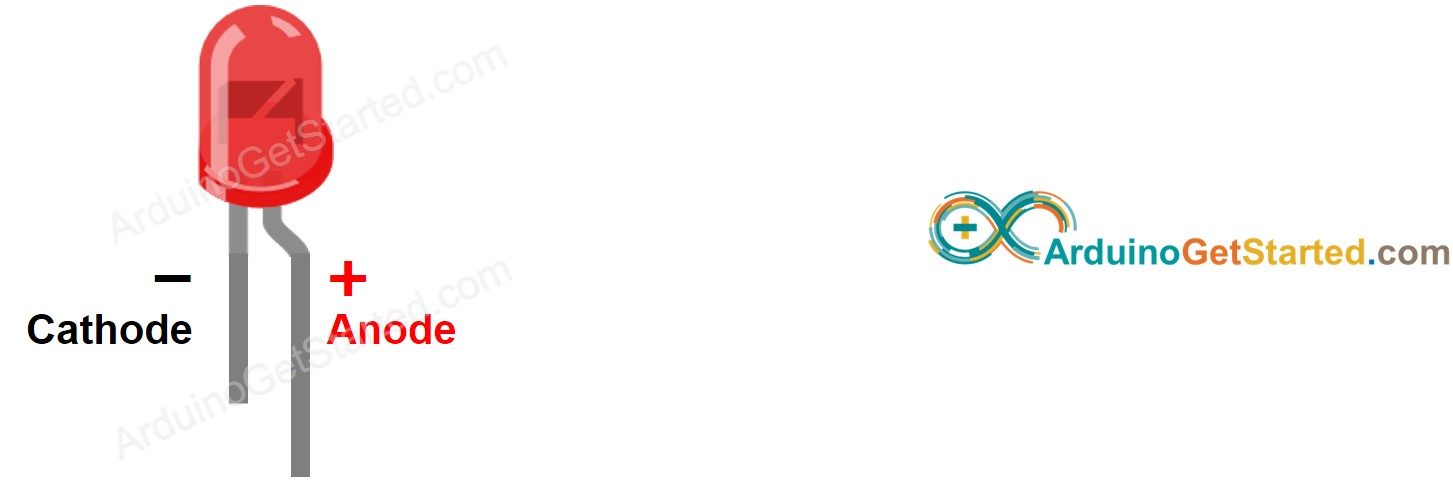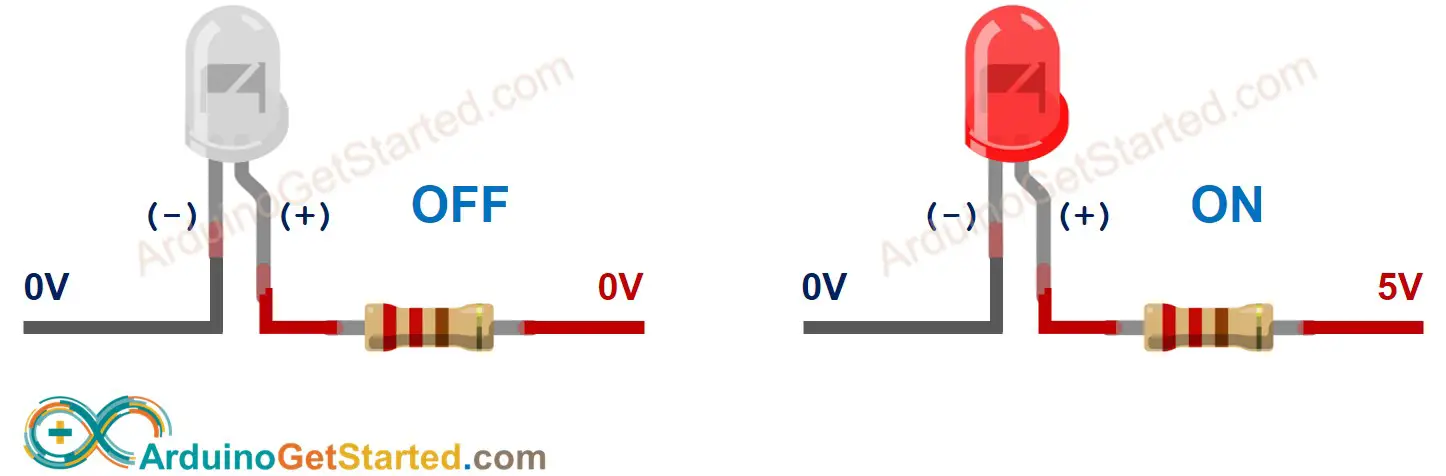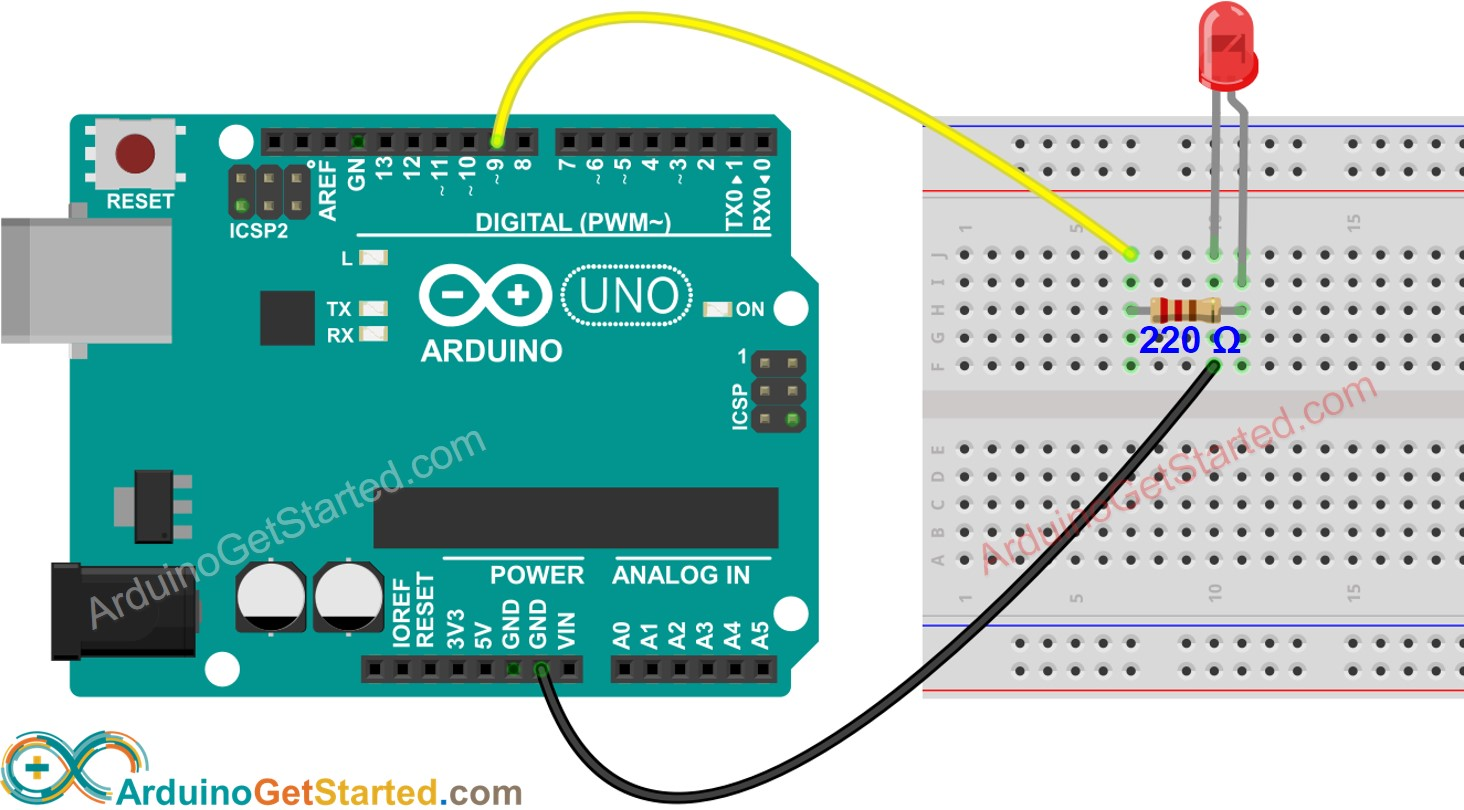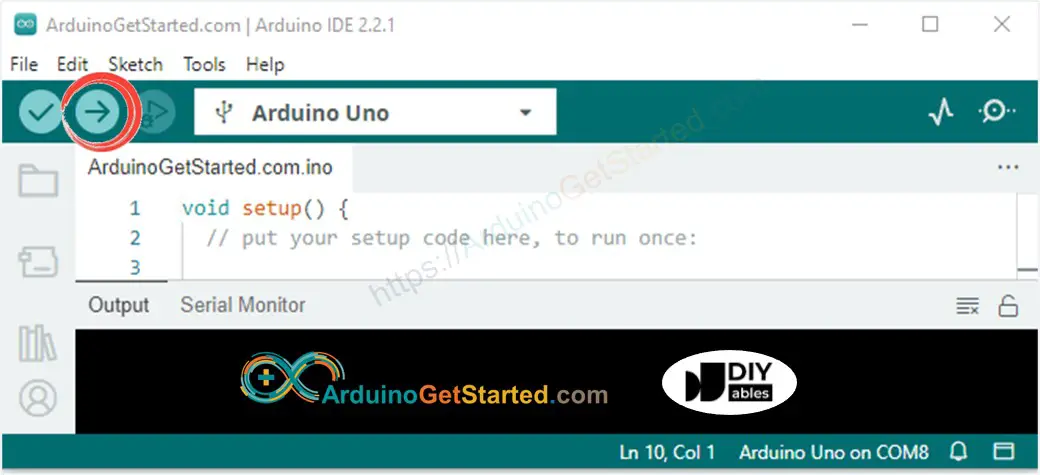# Arduino - LED - Blink

In this tutorial, we learn how to control LED with using Arduino, how to program for Arduino to turn LED on/off, and how to blink LED

## Hardware Required

Or you can buy the following sensor kit:

Please note: These are affiliate links. If you buy the components through these links, We may get a commission at no extra cost to you. We appreciate it.

### Pinout

LED includes two pins:

• Cathode(-) pin: needs to be connected to GND (0V)
• Anode(+) pin: is used to control LED's state### How It Works

After connecting the cathode(-) to GND:

• If connecting GND to the anode(+), LED is OFF.
• If connecting VCC to the anode(+), LED is ON.Besides, if generating a PWM signal to the anode(+), the brightness of LED is changed according to PWM value ( described in detail in this tutorial)

※ NOTE THAT:

• For most of LED, we need to use a resistor. The resistor can be placed between the anode(+) and VCC or between the cathode(-) and GND. The value of the resistor depends on the specification of the LED.
• Some kinds of LEDs have a built-in resistor. We may not need to use a resistor for those kinds of LEDs.

### Arduino - LED

When an Arduino's pin is configured as a digital output, the pin's voltage can be programmatically set to GND or VCC value.

By connecting the Arduino's pin to LED's anode(+) pin (via a resistor), we can programmatically control LED's state.

## Wiring Diagram

We are going to run through two examples:

• Example code controls the built-in LED on Arduino/Genuino UNO.
• Modifying Arduino Code controls the external LED.This image is created using Fritzing. Click to enlarge image

## How To Program

• Configure an Arduino's pin to the digital output mode by using pinMode() function. For example, pin 9:
pinMode(9, OUTPUT);
• Program the pin to GND to turn OFF led by using digitalWrite() function:
digitalWrite(9, LOW);
• Program the pin to VCC to turn ON led by using digitalWrite() function:
digitalWrite(9, HIGH);

## Arduino Code

### Quick Steps

• Connect Arduino to PC via USB cable
• Open Arduino IDE, select the right board and port
• Copy the below code and paste it to the Arduino IDE
/* * Created by ArduinoGetStarted.com * * This example code is in the public domain * * Tutorial page: https://arduinogetstarted.com/tutorials/arduino-led-blink */ // the setup function runs once when you press reset or power the board void setup() { // initialize digital pin LED_BUILTIN as an output. pinMode(LED_BUILTIN, OUTPUT); } // the loop function runs over and over again forever void loop() { digitalWrite(LED_BUILTIN, HIGH); // turn the LED on (HIGH is the voltage level) delay(1000); // wait for a second digitalWrite(LED_BUILTIN, LOW); // turn the LED off by making the voltage LOW delay(1000); // wait for a second }
• Click Upload button on Arduino IDE to upload code to Arduino• See the result: The built-in LED toggles between ON/OFF periodically every second.

### Code Explanation

Read the line-by-line explanation in comment lines of code!

※ NOTE THAT:

The above code uses the delay(). This function blocks Arduino from doing other tasks during the delay time. If your project requires to do some tasks, avoid blocking Arduino by using the non-blocking method for Arduino.

## Modifying Arduino Code

Modifying code to control the external LED

### Quick Steps

• Connect LED to Arduino via the above wiring diagram.
• Modify code:
• Change LED_BUILTIN to 9
• Change the delay from 1000 ms to 500 ms
/* * Created by ArduinoGetStarted.com * * This example code is in the public domain * * Tutorial page: https://arduinogetstarted.com/tutorials/arduino-led-blink */ // the setup function runs once when you press reset or power the board void setup() { // initialize digital pin 9 as an output. pinMode(9, OUTPUT); } // the loop function runs over and over again forever void loop() { digitalWrite(9, HIGH); // turn the LED on (HIGH is the voltage level) delay(500); // wait for 500 milliseconds digitalWrite(9, LOW); // turn the LED off by making the voltage LOW delay(500); // wait for 500 milliseconds }
• Click Upload button on Arduino IDE to upload code to Arduino
• See the result: LED switches between ON/OFF periodically. The LED blinks faster.

※ NOTE THAT:

This tutorial provides in-depth knowledge that helps you understand the working principle. To make it easy, you can use Arduino - LED library.

## Video Tutorial

We are considering to make the video tutorials. If you think the video tutorials are essential, please subscribe to our YouTube channel to give us motivation for making the videos.

## Challenge Yourself

• Connect LED to another pin of Arduino and change the blink time.
• Turn on/off LED by button. Hint: Refer to Arduino - Button.

Which pins on Arduino UNO can be used as an output pin to control LED?
• Pin 0 to pin 13
• Pin A0 to pin A5

※ NOTE THAT:

At a time, one pin can take only one task. If you already used a pin for another task (e.g, digital input, analog input, PWM, UART...), you should NOT use it as digital output to control LED. For example, if we use Serial.println() function, we should NOT use pin 0 and 1 for any other purpose because these pins are used for Serial.

## Extendability

This tutorial shows how to use the output pin of Arduino to control an LED. We can apply this code to control ON/OFF any devices, even big machines.

※ NOTE THAT:

for devices/machines that use a high power supply ( > 5v) and/or high-current consumption, we need to use a relay between output pin and devices/machines - see Arduino - Relay.

※ OUR MESSAGES